# The Vacuum Airship

Archimedes’ Principle states that the (upthrust) force on an object that is displacing a fluid is equal to the weight of the fluid displaced. For example, a cube with sides of one metre, fully submerged in water, will experience an upward force of 9810 newtons, as this is the weight of one cubic metre of water. The material that the object is made of has no effect on this force, so if the object weighs more than 9810 newtons it will sink, and if it weighs less than 9810 newtons it will float.

The upthrust force on an object therefore depends only on the relative densities of the object and the fluid it is displacing. A bigger difference means a bigger force.

Hydrogen is the least dense gas, at 0.0898 kilograms per metre cubed, but hydrogen is rarely used in airships as it is highly flammable and therefore dangerous. Hydrogen was used in the Hindenburg because  helium was difficult to produce and the United States, the only country with significant reserves, had banned its export. Helium has a density of 0.179 kg/m3 and therefore produces only 93% of the lift of hydrogen, but it is far, far safer.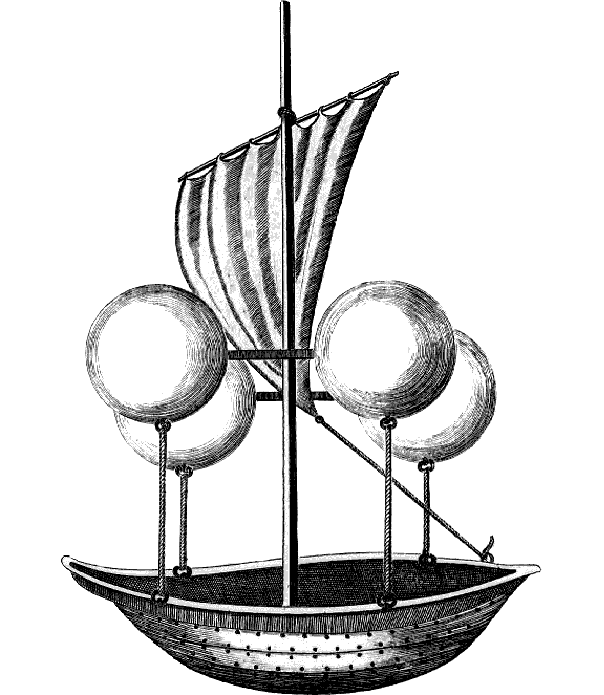Ideally, to create the maximum upthrust force, we would want our balloon or airship’s envelope to be filled with something with the lowest possible density. The lowest possible density would be a vacuum, the total absence of anything, which would create a lifting force of 12.7 newtons per cubic metre (as opposed to 11.0 N/m3 for helium).

The problem with using a vacuum to lift an airship, is how to contain the vacuum. If a difference in pressure exists between two regions, then a difference in force exists between those two regions. In the case of a vacuum airship being used on Earth’s surface, that force would be 101325 newtons per square metre, the equivalent of more than ten tonnes pushing down on every square metre. No material on Earth is strong enough to withstand this force without being so heavy as to negate the point of the vacuum lifting effect in the first place. In order to still have lifting capability and withstand the stresses involved, we can calculate the minimum required ratio of Young’s modulus to density, and this yields a figure of around 450000 Pa/(kg/m3)2. Unfortunately, even the strongest materials, like diamond, have ratios that are only one-fifth of this, so it looks like we won’t be creating vacuum airships any time soon.

# Galileo thermometer

Like most physicists, I have a soft spot for Galileo thermometers.A Galileo thermometer* works because the density of water changes as its temperature changes. The mass of a substance remains constant as it is heated (because the number of atoms doesn’t change) but because those atoms move faster the volume increases and therefore the density decreases, as shown in the graph for water below.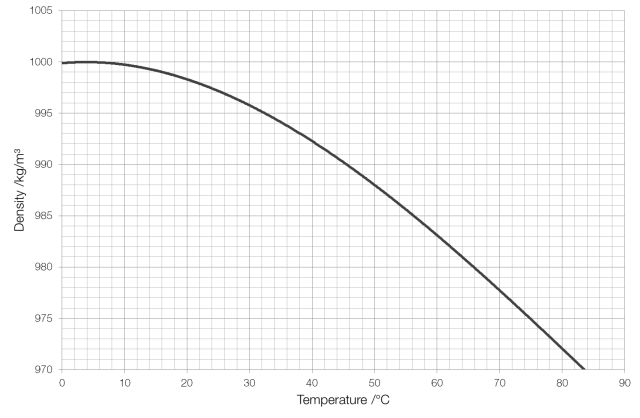As the water inside the thermometer is heated by its environment its density decreases and the more dense bubbles, that represent lower temperatures, are then more dense than the surrounding fluid and therefore sink. The Galileo thermometer is read by reading the temperature tag of the bubble closest to the middle of the cylinder.

The density of the bubbles inside the thermometer is set by altering the size of the metal tags attached to them: the bubbles that represent higher temperatures have smaller tags and therefore lower densities. (The overall density of the bubble does not change as it is heated because the overall density of the bubble depends only on its overall mass and overall volume, and as the liquid inside a bubble expands it merely compresses the air inside that bubble.)

* Not actually invented by Galileo, but by a group that included one of his students.

# Do bowling balls float?

The largest bowling ball allowed by USBC or FIQ has a circumference of 27 inches, equivalent to a diameter of 21.9 centimetres and a volume of 5447 cubic centimetres. Whilst the size of a bowling ball is fixed, its weight can vary, typically between six and sixteen pounds (2.7 to 7.3 kilograms).

With a fixed volume and a changing mass the density (mass per unit volume) of a bowling ball will change, and as a result some bowling balls float, and some sink.The low weight balls (including the 12 lb ball at a density of 999.6 kg/m3) all float. Only the 14 lb and 16 lb balls will sink.

# An interesting fact about the Eiffel Tower

I overheard one pupil tell another yesterday morning that:

“The air in a cylinder that contained the Eiffel Tower
would weigh more than the Eiffel Tower itself.”

It sounded feasible, but I wanted to check the maths.

The metal structure of the Eiffel Tower has a mass of 7300 tons, or 7.3 million kilograms. The design of the tower is very space-efficient: if you melted down the pure iron* that it is made of, it would occupy a volume of only 930m3, equivalent to a sphere with a radius of just six metres.The Eiffel Tower is 324 m tall and sits on a square base with sides of 125 m. It would therefore fit inside a cylinder with a radius of 88 metres, giving the cylinder a volume of just under eight million cubic metres. This much air would have a mass of 10 million kilograms.

So yes, that pupil was telling the truth. The air contained in a cylinder that could hold the Eiffel Tower would be 2.7 million kilograms heavier than the Eiffel Tower itself.

I am particularly fond of the Eiffel Tower as it lists, around its base, the names of seventy-two important French scientists, mathematicians and engineers, including Ampère, Arago, Becquerel, Daguerre, de Coulomb, Foucault, Fourier, Fresnel, Gay-Lussac, Lagrange, Laplace and Poisson.* More accurately, the tower is made from puddled iron, a type of wrought iron that has a density of 7850 kg/m3.

# Estimating density

It turns out that estimating density is something that pupils find really hard.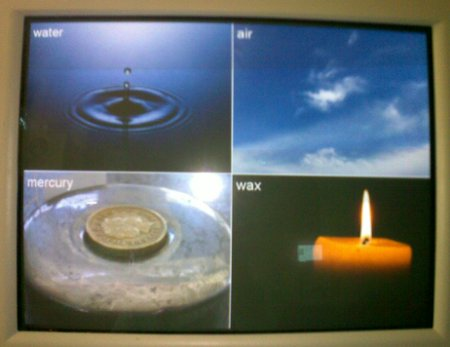I asked a class of pupils to estimate the density of four substances: air, water, mercury and wax. Before you read any further, try to estimate (in kilograms per metre cubed) the density of these four.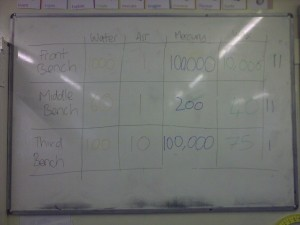In case you can’t read the photograph, their guesses (in kg/m3) were as follows:

• Water – 1000, 60, 100
• Air – 1, 1, 10
• Mercury – 100000, 200, 100000
• Wax – 10000, 40, 75

And the correct values: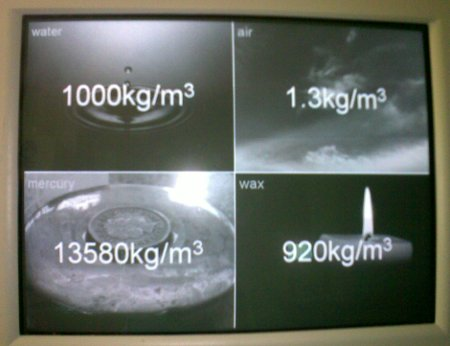They were much closer to the correct answer for air than for anything else, but probably only because we’ve discussed it before when looking at mass. Interestingly, taking the geometric mean of the suggested values for mercury gives a value of 12600kg/m3; very close to the correct value of 13580kg/m3, but this is almost certainly a fluke.

So why is estimating density so difficult?

Pupils have far less trouble estimating mass, and less trouble estimating volume (though converting from cm3 to m3 is something they find very taxing). I think the problem comes from a lack of reference points – they’ve all held a kilogram in their hand, and have used metre rulers frequently so can envision a box 1m×1m×1m. The main point of this exercise is to give them reference points. For example: if they calculate the density of a material to be 1200kg/m3 then they know it should sink in water, and something with a density of 1kg/m3 should be lighter than air.